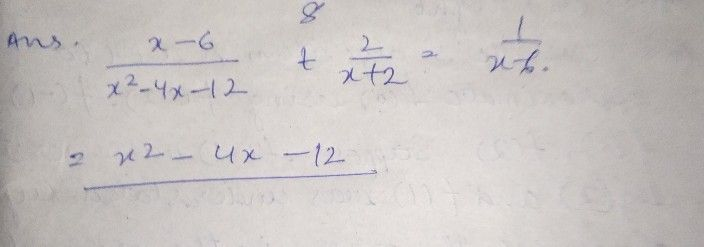Symbol
Problem$-$ Rational Equation $\dfrac {x-6} {x^{2}-4x-12}+\dfrac {2} {x+2}=\dfrac {1} {x-6}$ $1.$ Find the Least Common Denominator (LCD). $2.$ Multiply both sides of the equation by its the LCD. $3$ Apply the Distributive Property and then simplify. $\bar{4.} I$ Find all the possible values of $X.$ $x=10$ $5.$ Check each value by substituting into original equation and reject any extraneous $rO0t/s$
10th-13th grade
Calculus
Search count: 114
SolutionQanda teacher - Sheerin